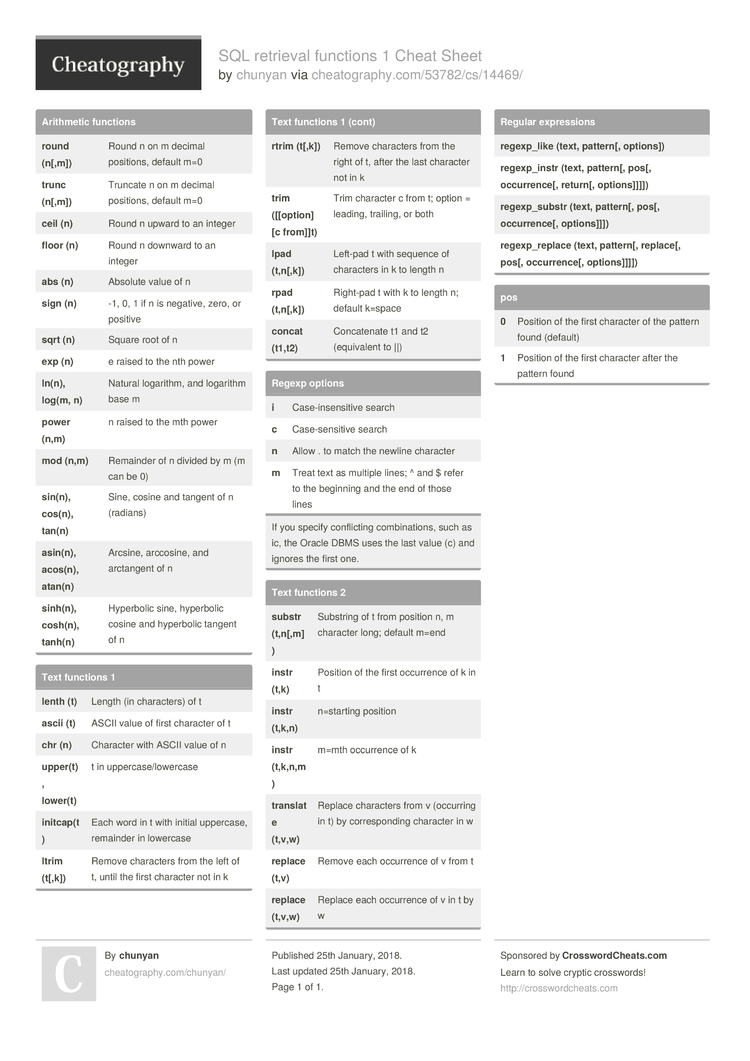# SQL retrieval functions 1 Cheat Sheet by chunyan

from the book: Beginning Oracle SQL for Oracle Database 12c

### Arithmetic functions

 round (n[,m]) Round n on m decimal positions, default m=0 trunc (n[,m]) Truncate n on m decimal positions, default m=0 ceil (n) Round n upward to an integer floor (n) Round n downward to an integer abs (n) Absolute value of n sign (n) -1, 0, 1 if n is negative, zero, or positive sqrt (n) Square root of n exp (n) e raised to the nth power ln(n), log(m, n) Natural logarithm, and logarithm base m power (n,m) n raised to the mth power mod (n,m) Remainder of n divided by m (m can be 0) sin(n), cos(n), tan(n) Sine, cosine and tangent of n (radians) asin(n), acos(n), atan(n) Arcsine, arccosine, and arctangent of n sinh(n), cosh(n), tanh(n) Hyperbolic sine, hyperbolic cosine and hyperbolic tangent of n

### Text functions 1

 lenth (t) Length (in charac­ters) of t ascii (t) ASCII value of first character of t chr (n) Character with ASCII value of n uppe­r(t), lower(t) t in upperc­ase­/lo­wercase init­cap­(t) Each word in t with initial uppercase, remainder in lowercase ltrim (t[,k]) Remove characters from the left of t, until the first character not in k rtrim (t[,k]) Remove characters from the right of t, after the last character not in k trim ([[option] [c from]]t) Trim character c from t; option = leading, trailing, or both lpad (t,n[,­k]) Left-pad t with sequence of characters in k to length n rpad (t,n[,­k]) Right-pad t with k to length n; default k=space concat (t1,t2) Concat­enate t1 and t2 (equiv­alent to ||)

### Regexp options

 i Case-i­nse­nsitive search c Case-s­ens­itive search n Allow . to match the newline character m Treat text as multiple lines; ^ and \$ refer to the beginning and the end of those lines
If you specify confli­cting combin­ations, such as ic, the Oracle DBMS uses the last value (c) and ignores the first one.

### Text functions 2

 substr (t,n[,­m]) Substring of t from position n, m character long; default m=end instr (t,k) Position of the first occurrence of k in t instr (t,k,n) n=starting position instr (t,k,n­,m) m=mth occurrence of k tran­slate (t,v,w) Replace characters from v (occurring in t) by corres­ponding character in w replace (t,v) Remove each occurrence of v from t replace (t,v,w) Replace each occurrence of v in t by w

### Regular expres­sions

 rege­xp_like (text, pattern[, option­s]) rege­xp_­instr (text, pattern[, pos[, occurrence[, return[, option­s]]­]]) rege­xp_­substr (text, pattern[, pos[, occurrence[, option­s]]]) rege­xp_­replace (text, pattern[, replace[, pos[, occurr­ence[, option­s]]­]])

### pos

 0 Position of the first character of the pattern found (default) 1 Position of the first character after the pattern found1 Page
//media.cheatography.com/storage/thumb/chunyan_sql-retrieval-functions-1.750.jpg

PDF (recommended)